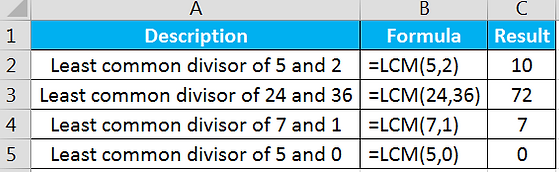# Math Function

1.1) Sum: This function add the number of series (Alt+=)

Syntax = SUM(number1, [number2],...)

Example1.2) Product: This function multiple all the number in given range

Syntax = PRODUCT(number1, [number2],..)

Example1.3)Power: This function returns the raised to a power

Syntax = POWER(number, power)

Example1.4) Mod: This function is returns the remainder after the number is divided by a divisor

Syntax = MOD(number, divisor)

Example1.5) Even: This function rounds the value with the nearest even integer

Syntax = EVEN(number)

Example1.6) Odd: This function rounds the value with the nearest odd integer

Syntax = ODD(number)

Example1.7) GCD: This function is returns the greatest common divisor

Syntax = GCD(number1, [number2],..)

Example1.8) LCM: This function is returns the least common divisor

Syntax = LCM(number1, [number2],..)

Example